# ELECTRIC FORCE AND ELECTRIC CHARGE

## 22.1. Introduction

Ordinary matter consists of atoms. Each atom consists of a nucleus, consisting of protons and neutrons, surrounded by a number of electrons. The masses of the electrons, protons and neutrons are listed in Table 22.1. Most of the mass of the atom is due to the mass of the nucleus.

 particle mass (kg) electron 9.11 x 10-31 proton 1.673 x 10-27 neutron 1.675 x 10-27

Table 22.1. Masses of the building blocks of atoms.

The diameter of the nucleus is between 10-15 and 10-14 m. The electrons are contained in a roughly spherical region with a diameter of about 2 x 10-10 m. In P121 it was shown that an object can only carry out circular motion if a radial force (directed towards the center of the circle) is present. Measurements of the velocity of the orbital electrons in an atom have shown that the attractive force between the electrons and the nucleus is significantly stronger than the gravitational force between these two objects. The attractive force between the electrons and the nucleus is called the electric force.

Experiments have shown that the electric force between two objects is proportional to the inverse square of the distance between the two objects. The electric force between two electrons is the same as the electric force between two protons when they are placed as the same distance. This implies that the electric force does not depend on the mass of the particle. Instead, it depends on a new quantity: the electric charge. The unit of electric charge q is the Coulomb (C). The electric charge can be negative, zero, or positive. Per definition, the electric charge on a glass rod rubbed with silk is positive. The electric charge of electrons, protons and neutrons are listed in Table 22.2. Detailed measurements have shown that the magnitude of the charge of the proton is exactly equal to the magnitude of the charge of the electron. Since atoms are neutral, the number of electrons must be equal to the number of protons.

The precise magnitude of the electric force that a charged particle exerts on another is given by Coulomb's law:

" The magnitude of the electric force that a particle exerts on another particle is directly proportional to the product of their charges and inversely proportional to the square of the distance between them. The direction of the force is along the line joining the particles. "

 particle charge (C) electron - 1.6 x 10-19 proton 1.6 x 10-19 neutron 0

Table 22.2. Electric charges of the building blocks of atoms

The electric force Fc can be written as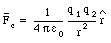(22.1)

where

q1 and q2 are the charges of particle 1 and particle 2, respectively

r is the distance between particle 1 and particle 2 (see Figure 22.1)

[epsilon]0 is he permittivity constant: [epsilon]0 = 8.85 x 10-12 C2/(N . m2)

This formula applies to elementary particles and small charged objects as long as their sizes are much less than the distance between them.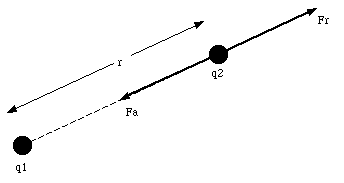Figure 22.1. Electric force between two charged objects.

An important difference between the electric force and the gravitational force is that the gravitational force is always attractive, while the electric force can be repulsive (Fc > 0), zero, or attractive (Fc < 0), depending on the charges of the particles. Table 22.3 lists the gravitational and the Coulomb force between electrons, protons and neutrons when they are separated by 1 x 10-10 m. This table shows clearly that the electric force dominates the motion of electrons in atoms. However, on a macroscopic scale, the gravitational force dominates. Since most macroscopic objects are neutral, they have an equal number of protons and electrons. The attractive force between the electrons in one body and the protons in the other body is exactly canceled by the repulsive force between the electrons in the two bodies.

Our discussion of the electric force will initially concentrate on those cases in which the charges are at rest or are moving very slowly. The electric force exerted under these circumstances is called the electrostatic force. If the charges are moving with a uniform velocity, they will experience both the electrostatic force and a magnetic force. The combined electrostatic and magnetic force is called the electromagnetic force.

 particle-particle Fg (N) Fc (N) electron - electron -5.5 x 10-51 2.3 x 10-8 electron - proton -1.0 x 10-47 - 2.3 x 10-8 electron - neutron -1.0 x 10-47 0 proton - proton - 1.9 x 10-44 2.3 x 10-8 proton - neutron - 1.9 x 10-44 0 neutron - neutron - 1.9 x 10-44 0

Table 22.3. The gravitational (Fg) and Coulomb (Fc) between the building blocks of atoms.

## 22.2. Charge Quantization and Charge Conservation

An important experiment in which the charge of small oil droplets was determined was carried out by Millikan (details of this experiment will be discussed in Chapter 23). Millikan discovered that the charge on the oil droplets was always a multiple of the charge of the electron (e, the fundamental charge). For example, he observed droplets with a charge equal to +/- e, +/- 2 e, +/- 3 e, etc., but never droplets with a charge equal to +/- 1.45 e, +/- 2.28 e, etc. The experiments strongly suggested that charge is quantized.

Another important property of charge is that charge a conserved quantity. No reaction has ever been found that creates or destroys charge. For example, the annihilation of an electron and an anti electron (positron) produces two photons: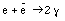(22.2)

This reaction does not violate conservation of charge. The initial charge is equal to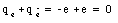(22.3)

Note that the charge of an antiparticle is opposite that of the particle. The final charge is equal to zero since photons are uncharged. The following reaction however violates conservation of electric charge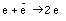(22.4)

This reaction has never been observed.

## 22.3. Conductors and Insulators

A conductor is a material that permits the motion of electric charge through its volume. Examples of conductors are copper, aluminum and iron. An electric charge placed on the end of a conductor will spread out over the entire conductor until an equilibrium distribution is established. In contrast, electric charge placed on an insulator stays in place: an insulator (like glass, rubber and Mylar) does not permit the motion of electric charge.Figure 22.2. Induction of Charge on Metal Sphere.

The properties of a conductor are a result of the presence of free electrons in the material. These electrons are free to move through the entire volume of the conductor. Because of the free electrons, the charge distribution of a conductor can be changed by the presence of external charges. For example, the metal sphere shown in Figure 22.2 is initially uncharged. This implies that the free electrons (and positive ions) are distributed uniformly over its surface. If a rod with a positive charge is placed in the vicinity of the sphere, it will produce an attractive force on the free electrons. As a consequence of this attractive force the free electrons will be redistributed, and the top of the conductor will get a negative charge (excess of electrons). Since the number of free electrons on the sphere is unchanged, the bottom of the sphere will have a deficit of free electrons (and will have a positive charge). The positive ions are bound to the lattice of the material, and their distribution is not affected by the presence of the charged rod. If we connect the bottom of the sphere to ground (a source or drain of electrons) electrons will be attracted by the positive charge. The number of electrons on the sphere will increase, and the sphere will have a net negative charge. If we break the connection to the ground before removing the charged rod, we are left with a negative charge on the sphere. If we first remove the charged rod, the excess of electrons will drain to the ground, and the sphere will become uncharged.

Send comments, questions and/or suggestions via email to wolfs@nsrl.rochester.edu and/or visit the home page of Frank Wolfs.